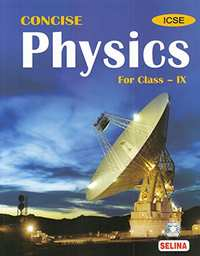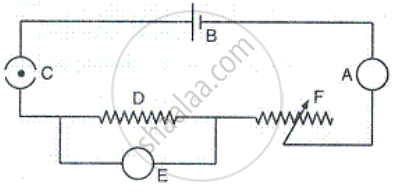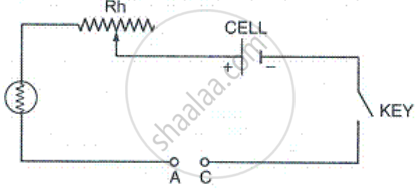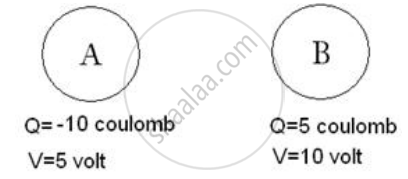# Selina solutions for Concise Physics Class 9 ICSE chapter 9 - Current Electricity [Latest edition]

#### Chapters## Chapter 9: Current Electricity

Exercise 9 (A)Exercise 9 (B)Exercise 9 (C)
Exercise 9 (A) [Page 193]

### Selina solutions for Concise Physics Class 9 ICSE Chapter 9 Current Electricity Exercise 9 (A) [Page 193]

Exercise 9 (A) | Q 1 | Page 193

Name one D.C. source and one A.C. source.

Exercise 9 (A) | Q 2 | Page 193

Distinguish between D.C. and A.C.

Exercise 9 (A) | Q 3 | Page 193

What is an electric cell?

Exercise 9 (A) | Q 4 | Page 193

What transformation of energy takes place when current is drawn from a cell?

Exercise 9 (A) | Q 5 | Page 193

Name the constituents of a cell.

Exercise 9 (A) | Q 6 | Page 193

State the two kinds of cell. Give one example of each.

Exercise 9 (A) | Q 7 | Page 193

What is a primary cell? Name two such cells.

Exercise 9 (A) | Q 8 | Page 193

What is a secondary cell? Name one such cell.

Exercise 9 (A) | Q 9 | Page 193

State three differences between the primary and secondary cells.

Exercise 9 (A) | Q 10 | Page 193

What do you understand by the term current? State and define its S.I. unit.

Exercise 9 (A) | Q 11 | Page 193

How much is the charge on an electron?

Exercise 9 (A) | Q 12 | Page 193

n electrons flow through a cross section of a conductor in time t. If charge on an electron is e, then write an expression for the current in the conductor.

Exercise 9 (A) | Q 13 | Page 193

Name the instrument used to control the current in an electric circuit.

Exercise 9 (A) | Q 14 | Page 193

In the electric circuit shown in Figure, label the parts A, B, C, D, E, and F. State the function of each part. Show in the diagram the direction of flow of current.Exercise 9 (A) | Q 15 | Page 193

What is the function of a key (or switch) in an electric circuit?

Exercise 9 (A) | Q 16.1 | Page 193

Write symbol and state function of following component in an electric circuit : key

Exercise 9 (A) | Q 16.2 | Page 193

Write symbol and state function of following component in an electric circuit :

cell

Exercise 9 (A) | Q 16.3 | Page 193

Write symbol and state function of following component in an electric circuit :

rheostat

Exercise 9 (A) | Q 16.4 | Page 193

Write symbol and state function of following component in an electric circuit :

ammeter

Exercise 9 (A) | Q 16.5 | Page 193

Write symbol and state function of following component in an electric circuit :

voltmeter

Exercise 9 (A) | Q 17 | Page 193

(a) Complete the circuit given in Fig. by inserting between the terminals  A and C. an ammeter.

(b) In the diagram, mark the polarity at the terminals of ammeter and indicate clearly the direction of flow of current in the circuit when the circuit is complete.

(c) Name and state the purpose of Rh in the circuit.Exercise 9 (A) | Q 18 | Page 193

What are conductors and insulators of electricity? Give two examples of each.

Exercise 9 (A) | Q 19 | Page 193

Select conductors of electricity from the following: Copper wire, silk thread, pure water, acidulated water, human body, glass, mercury.

Exercise 9 (A) | Q 20 | Page 193

State two differences between a conductor and an insulator of electricity.

Exercise 9 (A) | Q 21 | Page 193

Distinguish between a closed circuit and an open circuit, with the use of suitable labelled diagrams.

Exercise 9 (A) | Q 22 | Page 193

Write the condition required for a circuit to be a closed circuit.

Exercise 9 (A) [Page 193]

### Selina solutions for Concise Physics Class 9 ICSE Chapter 9 Current Electricity Exercise 9 (A) [Page 193]

Exercise 9 (A) | Q 1 | Page 193

A cell is used to :

• measure current in a circuit

• provide current in a circuit

• check current in a circuit

• prevent current in a circuit.

Exercise 9 (A) | Q 2 | Page 193

The unit of current is :

• ampere

• volt

• ohm

• coulomb

Exercise 9 (A) | Q 3 | Page 193

The insulator of electricity is :

• Copper

• Acidulated water

• Human body

• Silk

Exercise 9 (A) [Page 194]

### Selina solutions for Concise Physics Class 9 ICSE Chapter 9 Current Electricity Exercise 9 (A) [Page 194]

Exercise 9 (A) | Q 1 | Page 194

A charge 0.5 C passes through a cross section of a conductor in 5 s . Find the current .

Exercise 9 (A) | Q 2 | Page 194

A current of 1.5 A flows through a conductor for 2.0 s . What amount of charge passes through the conductor ?

Exercise 9 (A) | Q 3 | Page 194

When starter motor of a car is switched on for 0.8 s . a charge 24 C passes through the coil of the motor . Calculate the current in the coil .

Exercise 9 (B) [Page 198]

### Selina solutions for Concise Physics Class 9 ICSE Chapter 9 Current Electricity Exercise 9 (B) [Page 198]

Exercise 9 (B) | Q 1 | Page 198

Figure. below shows two conductors A and B. Their charges and potentials are given in diagram. State the direction of (i) flow of electrons and (ii) flow of current, when both the conductors are joined by a metal wire.Exercise 9 (B) | Q 2 | Page 198

How is the direction of flow of current between the two charged conductors determined by their potentials?

Exercise 9 (B) | Q 3 | Page 198

Explain the concept of electric potential difference in terms of work done in transferring the charge.

Exercise 9 (B) | Q 4 | Page 198

Define the term potential difference?

Exercise 9 (B) | Q 5 | Page 198

State and define the S.I. unit of potential difference.

Exercise 9 (B) | Q 6 | Page 198

'The potential difference between two conductors is 1 volt'. Explain the meaning of this statement.

Exercise 9 (B) | Q 7 | Page 198

What do you understand by the term resistance?

Exercise 9 (B) | Q 8 | Page 198

Explain why does a metal wire when connected to a cell offer resistance to the flow of current.

Exercise 9 (B) | Q 9 | Page 198

State and define the S.I. unit of resistance.

Exercise 9 (B) | Q 10 | Page 198

State Ohm's law.

Exercise 9 (B) | Q 11 | Page 198

How are the potential difference (V), current (l) and resistance (R) related?

Exercise 9 (B) | Q 12 | Page 198

'The resistance of a wire is 1 ohm'. Explain the meaning of this statement.

Exercise 9 (B) | Q 13 | Page 198

How is the current flowing in a conductor changed if the resistance of conductor is doubled keeping the potential difference across it the same?

Exercise 9 (B) | Q 14 | Page 198

State three factors on which the resistance of a wire depends. Explain how the resistance depends on the factors stated by you.

Exercise 9 (B) | Q 15 | Page 198

How is the resistance of a wire affected if its (a) length is doubled, (b) radius is doubled?

Exercise 9 (B) | Q 16 | Page 198

State whether the resistance of filament of a bulb will decrease, remain unchanged or increase when it glows.

Exercise 9 (B) | Q 17.1 | Page 198

Name the physical quantity of which the unit is volt

Exercise 9 (B) | Q 17.2 | Page 198

Name the physical quantity of which the unit is coulomb

Exercise 9 (B) | Q 17.3 | Page 198

Name the physical quantity of which the unit is ohm

Exercise 9 (B) | Q 17.4 | Page 198

Name the physical quantitiy of which the unit is ampere

Exercise 9 (B) [Page 198]

### Selina solutions for Concise Physics Class 9 ICSE Chapter 9 Current Electricity Exercise 9 (B) [Page 198]

Exercise 9 (B) | Q 1 | Page 198

Current in a circuit flows :

•  In direction from high potential to low potential

• In direction from low potential to high potential

• In direction of flow of electrons

• In any direction.

Exercise 9 (B) | Q 2 | Page 198

The unit of potential difference is :

• ampere

• volt

• ohm

• coulomb

Exercise 9 (B) | Q 3 | Page 198

On increasing the resistance in a circuit, the current in it :

• Decreases

• Increases

• Remains unchanged

• Nothing can be said.

Exercise 9 (B) [Page 198]

### Selina solutions for Concise Physics Class 9 ICSE Chapter 9 Current Electricity Exercise 9 (B) [Page 198]

Exercise 9 (B) | Q 1 | Page 198

In transferring 1.5 C charge through a wire, 9 J of work is needed. Find the potential difference across the wire.

Exercise 9 (B) | Q 2 | Page 198

A cell of potential difference 12 V is connected to a bulb. The resistance of filament of bulb when it glows is 24Ω. Find the current drawn from the cell.

Exercise 9 (B) | Q 3 | Page 198

A bulb draws current 1.5 A at 6.0 V. Find the resistance of filament of bulb while glowing.

Exercise 9 (B) | Q 4 | Page 198

A current 0.2 A flows in a wire of resistance 15Ω. Find the potential difference across the ends of the wire.

Exercise 9 (C) [Page 199]

### Selina solutions for Concise Physics Class 9 ICSE Chapter 9 Current Electricity Exercise 9 (C) [Page 199]

Exercise 9 (C) | Q 1 | Page 199

What is meant by the efficient use of energy?

Exercise 9 (C) | Q 2 | Page 199

State two ways to save the energy.

Exercise 9 (C) | Q 3 | Page 199

How does the proper insulation of home save energy?

Exercise 9 (C) | Q 4 | Page 199

Which of the following device is most efficient for the lighting purpose?

LED, CFL, fluorescent tube light, Electric bulb.

Exercise 9 (C) | Q 5 | Page 199

Give an example to explain that the use of modem eco-friendly technology is more efficient and less polluting.

Exercise 9 (C) | Q 6 | Page 199

Describe three ways for the efficient use of energy.

Exercise 9 (C) | Q 7 | Page 199

What social initiatives must be taken for the sensitive use of energy?

Exercise 9 (C) [Page 199]

### Selina solutions for Concise Physics Class 9 ICSE Chapter 9 Current Electricity Exercise 9 (C) [Page 199]

Exercise 9 (C) | Q 1 | Page 199

The most non-polluting and efficient lighting device is :

• CFL

• LED

• Fluorescent light

• Electric bulb

Exercise 9 (C) | Q 2 | Page 199

IEA is the short form of :

• Indian Energy Association

• International Energy Agency

## Chapter 9: Current Electricity

Exercise 9 (A)Exercise 9 (B)Exercise 9 (C)## Selina solutions for Concise Physics Class 9 ICSE chapter 9 - Current Electricity

Selina solutions for Concise Physics Class 9 ICSE chapter 9 (Current Electricity) include all questions with solution and detail explanation. This will clear students doubts about any question and improve application skills while preparing for board exams. The detailed, step-by-step solutions will help you understand the concepts better and clear your confusions, if any. Shaalaa.com has the CISCE Concise Physics Class 9 ICSE solutions in a manner that help students grasp basic concepts better and faster.

Further, we at Shaalaa.com provide such solutions so that students can prepare for written exams. Selina textbook solutions can be a core help for self-study and acts as a perfect self-help guidance for students.

Concepts covered in Concise Physics Class 9 ICSE chapter 9 Current Electricity are Simple Electric Circuit Using an Electric Cell and a Bulb to Introduce the Idea of Current, Insulators and Conductors, Closed and Open Circuits, Direction of Current (Electron Flow and Conventional), Social Initiatives - Improving Efficiency of Existing Technologies and Introducing New Eco-friendly Technologies. Creating Awareness and Building Trends of Sensitive Use of Resources and Products.

Using Selina Class 9 solutions Current Electricity exercise by students are an easy way to prepare for the exams, as they involve solutions arranged chapter-wise also page wise. The questions involved in Selina Solutions are important questions that can be asked in the final exam. Maximum students of CISCE Class 9 prefer Selina Textbook Solutions to score more in exam.

Get the free view of chapter 9 Current Electricity Class 9 extra questions for Concise Physics Class 9 ICSE and can use Shaalaa.com to keep it handy for your exam preparation